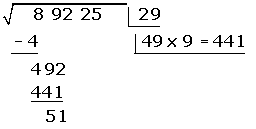Chapters## 1

If the radicand has more than two figures, separate the figures in groups of two starting with the right.## 2

Calculate the square root of the first group of figures from the left.

What number squared gives 8?

8 is not a perfect square but it is between two perfect squares: 4 and 9, then take the square root of the perfect square default: 2, and place it in the box.## 3

The square of the result obtained is subtracted from the first group of figures that appear in the radicand.The square of 2 is 4, subtract it to 8 and get 4.

It goes down to 92, the amount of operable radicand: 492.

## 4

Behind the remainder put the next group of figures of radicand, separating the number formed by the first figure on the right and dividing the remainder by twice the previous root.49 : 4 > 9, take as a result, 9.

## 5

The quotient that is obtained is placed behind the double of the root, by multiplying the number formed by it, and subtracting the amount of radicand operable.If a higher value was obtained to the amount operable of the radicand, 8, 7... would have been tested until a lower value is found.## 6

The quotient obtained is the second figure of the root.## 7

Go down the next pair of digits and repeat the steps above.As 5301 > 5125, test by 8.Raise 8 to the root.## 8

Test of the square root.

For the result is correct, must be satisfied:

Radicand = (Root) ² + Remainder

89,225 = 298² + 421

Did you like the article?(No Ratings Yet)Loading...

Emma

I am passionate about travelling and currently live and work in Paris. I like to spend my time reading, gardening, running, learning languages and exploring new places.

Did you like
this resource?

Bravo!